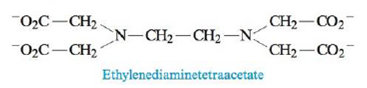Chapter 16, Problem 87AE

Chapter
Section
Textbook Problem

# Calculate the concentration of Pb2+ in each of the following.a. a saturated solution of Pb(OH)2, Ksp = 1.2 × 10−15b. a saturated solution of Pb(OH)2 buffered at pH = 13.00c. Ethylenediaminetetraacetate (EDTA4−) is used as a complexing agent in chemical analysis and has the following structure:Solutions of EDTA4− are used to treat heavy metal poisoning by removing the heavy metal in the form of a soluble complex ion. The reaction of EDTA4− with Pb2+ is Pb 2 + ( a q )   +   E D T A 4 −   ( a q )   ⇌   P b E D T A 2 − ( a q )   K   =   1.1   ×   10 18 Consider a solution with 0.010 mole of Pb(NO3)2 added to 1.0 L of an aqueous solution buffered at pH = 13.00 and containing 0.050 M Na4.EDTA. Does Pb(OH)2 precipitate from this solution?

(a)

Interpretation Introduction

Interpretation: The concentration of Pb2+ is to be calculated in each of the given cases.

Concept introduction: Solubility is defined as the maximum amount of solute that can dissolve at a certain amount of solvent at certain temperature. The solubility product, Ksp is the equilibrium constant that is applied when salt partially dissolve in a solvent. The solubility product of dissociation of AxBy is calculated by the formula,

Ksp=[A]x[B]y

Explanation

Explanation

To determine: The concentration of Pb2+ is to be calculated in a saturated solution of Pb(OH)2 .

The solubility of Pb2+ is 6.7×10-6mol/L_ .

The reaction at equilibrium is,

Pb(OH)2(s)Pb2+(aq)+2OH(aq)

The solubility product of dissociation of Pb(OH)2 is calculated by the formula,

Ksp=[Pb2+][OH]

The solubility of Pb(OH)2 can be calculated from the concentration of its ions at equilibrium.

It is assumed that smol/L of solid is dissolved to reach the equilibrium. The meaning of 1:2 stoichiometry of salt is,

smol/LPb(OH)2smol/LPb2++2smol/OH

Formula

The solubility product of Pb(OH)2 is calculated as,

Ksp=[Pb2+][OH]2

Where,

• Ksp is solubility product

(b)

Interpretation Introduction

Interpretation: The concentration of Pb2+ is to be calculated in each of the given cases.

Concept introduction: Solubility is defined as the maximum amount of solute that can dissolve at a certain amount of solvent at certain temperature. The solubility product, Ksp is the equilibrium constant that is applied when salt partially dissolve in a solvent. The solubility product of dissociation of AxBy is calculated by the formula,

Ksp=[A]x[B]y

(c)

Interpretation Introduction

Interpretation: The concentration of Pb2+ is to be calculated in each of the given cases.

Concept introduction: Solubility is defined as the maximum amount of solute that can dissolve at a certain amount of solvent at certain temperature. The solubility product, Ksp is the equilibrium constant that is applied when salt partially dissolve in a solvent. The solubility product of dissociation of AxBy is calculated by the formula,

Ksp=[A]x[B]y

### Still sussing out bartleby?

Check out a sample textbook solution.

See a sample solution

#### The Solution to Your Study Problems

Bartleby provides explanations to thousands of textbook problems written by our experts, many with advanced degrees!

Get Started

#### Find more solutions based on key concepts